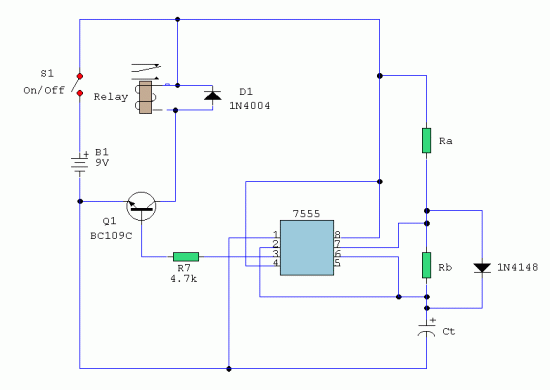## Asymmetric Timer

Description:
A timer circuit with independent mark and space periods.Notes:
A simple astable timer made with the 555, the mark (on) and space (off) values may be set independently. The timing chain consists of resistors Ra, Rb and capacitor Ct. The capacitor, Ct charges via Ra which is in series with the 1N4148 diode. The discharge path is via Rb into into pin 7 of the IC. Both halves of the timing period can now be set independently.

The charge time (output high) is calculated by:

T(on) = 0.7 Ra Ct

The discharge time (output low) is calculated by:

T(off) = 0.7 Rb Ct

Please note that the formula for T(on) ignores the series resistance and forward voltage of the 1N4148 and is therefore approximate, but T(off) is not affected by D1 and is therefore precise